Holonomic system

A system of material points that is either not constrained by any constraint or constrained only by geometric constraints. The latter impose restrictions on the positions of the points of the system and may be represented by relations of the type(1)

Hereis the time,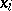are the Cartesian coordinates of the point andis the number of points in the system. If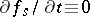, the constraints are said to be stationary; otherwise they are called non-stationary. Any position of the system for which the coordinates of the points obey equations (1) is called possible for the given moment. The constraints (1) impose restrictions not only on the positions, but also on the velocities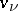and on the accelerations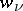of the points: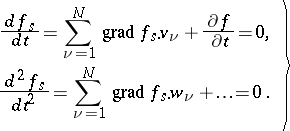(2)

The velocities and accelerations satisfying equations (2) are said to be kinematically possible in a given position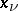of the system for a given moment of time. Infinitesimal displacementswhich satisfy conditions of the type(3)

are the possible (virtual) displacements of the system, as distinct from the real displacements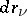performed by the system within the time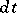under the effect of forces applied to it and satisfying conditions of the type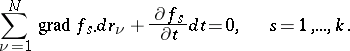(4)

For stationary constraints real displacements are found among the possible displacements, while for non-stationary constraints they are not found among the possible displacements, in general. Possible displacements are useful for converting a holonomic system from one position of the system which is possible for a givento another infinitely-close position which is possible at the same moment.

The number of independent variations of the points of the system is said to be the number of its degrees of freedom; for a holonomic system this coincides with the numberof independent arbitrary parameters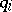with the aid of which equations (1) may be represented in the form of relations of the type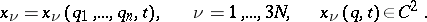(5)

The parametersare called generalized, or Lagrangian, coordinates of the system; they are called holonomic coordinates, in distinction from non-holonomic, or quasi-, coordinates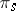, introduced by non-integrable relations of the type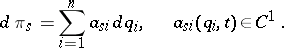(6)

Constraints that can be analytically expressed by (1) are said to be retaining, or two-sided, in distinction from non-retaining, or one-sided, constraints, which can be expressed by inequalities of the type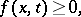and impose the following conditions on the possible displacements: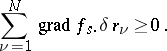The possible displacements of a system with two-sided constraints are reversible; among the possible displacements of a system with one-sided constraints there are irreversible ones .

The motions of holonomic systems are described by the Lagrange equations (in mechanics) (of the first and second kinds), by the Hamilton equations in Lagrangian coordinates and impulses, the Appell equations, the Poincaré equations or the Chetaev equations in Lagrangian coordinates and quasi-coordinates.